Courses

# RD Sharma Solutions - Ex-23.4, Data Handling II Central Values, Class 7, Math Class 7 Notes | EduRev

## Class 7: RD Sharma Solutions - Ex-23.4, Data Handling II Central Values, Class 7, Math Class 7 Notes | EduRev

The document RD Sharma Solutions - Ex-23.4, Data Handling II Central Values, Class 7, Math Class 7 Notes | EduRev is a part of the Class 7 Course RD Sharma Solutions for Class 7 Mathematics.
All you need of Class 7 at this link: Class 7

Question 1:

Find the mode and median of the data: 13, 16, 12, 14, 19, 12, 14, 13, 14
By using the empirical relation also find the mean.

Arranging the data in ascending order such that same numbers are put together, we get:
12,12,13,13, 14,14,14, 16, 19
Here, n = 9.
∴ Median = Value of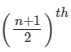observation = Value of the 5th observation = 14.
Here, 14 occurs the maximum number of times, i.e., three times. Therefore, 14 is the mode of the data.

Now,
Mode = 3 Median - 2 Mean
⇒ 14 = 3 x 14 - 2 Mean
⇒2 Mean  = 42 - 14 = 28
⇒ Mean = 28 ÷ 2 = 14.

#### Question 2:

Find the median and mode of the data: 35, 32, 35, 42, 38, 32, 34

Arranging the data in ascending order such that same numbers are put together, we get:

32, 32, 34,35,35, 38,42.

Here, n = 7
∴ Median = Value ofobservation = Value of the 4th observation = 35.
Here, 32 and 35, both occur twice. Therefore, 32 and 35 are the two modes.

#### Question 3:

Find the mode of the data: 2, 6, 5, 3, 0, 3, 4, 3, 2, 4, 5, 2, 4

Arranging the data in ascending order such that same values are put together, we get:

0, 2, 2, 2, 3, 3,3,4,4,4,5,5,6.

Here, 2,3 and 4 occur three times each. Therefore, 2 ,3 and 4 are the three modes.

Alternate Solution
Arranging the data in the form of a frequency table, we have:

 Values Tally Bars Frequency 0 ∣ 1 2 ∣∣∣ 3 3 ∣∣∣ 3 4 ∣∣∣ 3 5 ∣∣ 2 6 ∣ 1 Total 13

Clearly, the values 2,3 and 4 occur the maximum number of times, i.e., three times.
Hence, the mode is 2,3 and 4.

#### Question 4:

The runs scored in a cricket match by 11 players are as follows:
6, 15, 120, 50, 100, 80, 10, 15, 8, 10, 10
Find the mean, mode and median of this data.

Arranging the data in ascending order such that same values are put together, we get:

6,8,10,10,10,15,15,50,80,100,120.

Here, n = 11
∴ Median = Value ofobservation = Value of the 6th observation = 15.
Here, 10 occurs three times. Therefore, 10 is the mode of the given data.
Now,
Mode = 3 Median - 2 Mean
⇒ 10 = 3 x 15 - 2 Mean
⇒2 Mean  = 45 - 10 = 35
⇒ Mean = 35 ÷ 2 = 17.5.

#### Question 5:

Find the mode of the following data:
12, 14, 16, 12, 14, 14, 16, 14, 10, 14, 18, 14

Arranging the data in ascending order such that same values are put together, we get:

10,12,12,14,14,14,14,14,14, 16, 16, 18.

Here, clearly, 14 occurs the most number of times.
Therefore, 14 is the mode of the given data.

Alternate solution:
Arranging the data in the form of a frequency table, we get:

 Values Tally Bars Frequency 10 ∣ 1 12 ∣∣ 2 14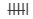6 16 ∣∣ 2 18 ∣ 1 Total 12

Clearly, 14 has maximum frequency. So, the mode of the given data is 14.

#### Question 6:

Heights of 25 children (in cm) in a school are as given below:
168, 165, 163, 160, 163, 161, 162, 164, 163, 162, 164, 163, 160, 163, 163, 165, 163, 162, 163, 164, 163, 160, 165, 163, 162
What is the mode of heights?
Also, find the mean and median.

Arranging the data in tabular form, we get:

 Height of Children (cm) Tally Bars Frequency 160 ∣∣∣ 3 161 ∣ 1 162 ∣∣∣∣ 4 163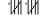10 164 ∣∣∣ 3 165 ∣∣∣ 3 168 ∣ 1 Total 25

Here, n = 25
∴ Median = Value ofobservation = Value of the 13th observation = 163 cm.
Here, clearly, 163 cm occurs the most number of times. Therefore, the mode of the given data is 163 cm.
Now,
Mode = 3 Median - 2 Mean
⇒ 163 = 3 x 163 - 2 Mean
⇒2 Mean  =  326
⇒ Mean = 326 ÷ 2 = 163 cm.

#### Question 7:

The scores in mathematics test (out of 25) of 15 students are as follows:
19, 25, 23, 20, 9, 20, 15, 10, 5, 16, 25, 20, 24, 12, 20
Find the mode and median of this data. Are they same?

Arranging the data in ascending order such that same values are put together, we get:

5,9,10,12,15,16, 19, 20, 20, 20, 20,  23, 24, 25, 25.
Here, n = 15
∴ Median = Value ofobservation = Value of the 8th observation = 20.
Here, clearly, 20 occurs the most number of times, i.e., 4 times. Therefore, the mode of the given data is 20.
Yes, the median and mode of the given data are the same.

#### Question 8:

Calculate the mean and median for the folllowing data:

 Marks : 10 11 12 13 14 16 19 20 Number of students : 3 5 4 5 2 3 2 1

Using empirical formula, find its mode.

Calculation of Mean

 Marks (xi) 10 11 12 13 14 16 19 20 Total Number of Students (fi) 3 5 4 5 2 3 2 1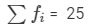fixi 30 55 48 65 28 48 38 20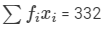Mean  =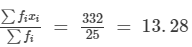Here, n = 25, which is an odd number. Therefore,
Median = Value ofobservation = the 13th observation = 13.
Now,
Mode = 3 Median - 2 Mean

⇒Mode = 3 x 13 - 2 x (13.28)
⇒Mode = 39 - 26.56
⇒Mode = 12.44.

#### Question 9:

The following table shows the weights of 12 persons.

 Weight (in kg): 48 50 52 54 58 Number of persons: 4 3 2 2 1

Find the median and mean weights. Using empirical relation, calculate its mode.

Calculation of Mean

 Weight (xi) 48 50 52 54 58 Total Number of Persons (fi) 4 3 2 2 1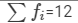fixi 192 150 104 108 58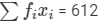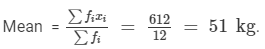Here, n = 12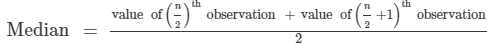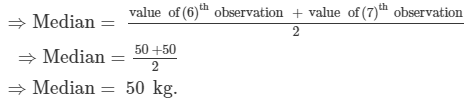Now,
Mode = 3 Median - 2 Mean
⇒ Mode = 3 x 50 - 2 x 51
⇒Mode  = 150 - 102
⇒ Mode = 48 kg.
Thus, Mean = 51 kg, Median = 50 kg and Mode = 48 kg.

The document RD Sharma Solutions - Ex-23.4, Data Handling II Central Values, Class 7, Math Class 7 Notes | EduRev is a part of the Class 7 Course RD Sharma Solutions for Class 7 Mathematics.
All you need of Class 7 at this link: Class 7Use Code STAYHOME200 and get INR 200 additional OFF Use Coupon Code
All Tests, Videos & Notes of Class 7: Class 7

### Up next### Top Courses for Class 7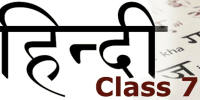## RD Sharma Solutions for Class 7 Mathematics

97 docs

### Up next### Top Courses for Class 7Track your progress, build streaks, highlight & save important lessons and more!

,

,

,

,

,

,

,

,

,

,

,

,

,

,

,

,

,

,

,

,

,

,

,

,

,

,

,

,

,

,

;